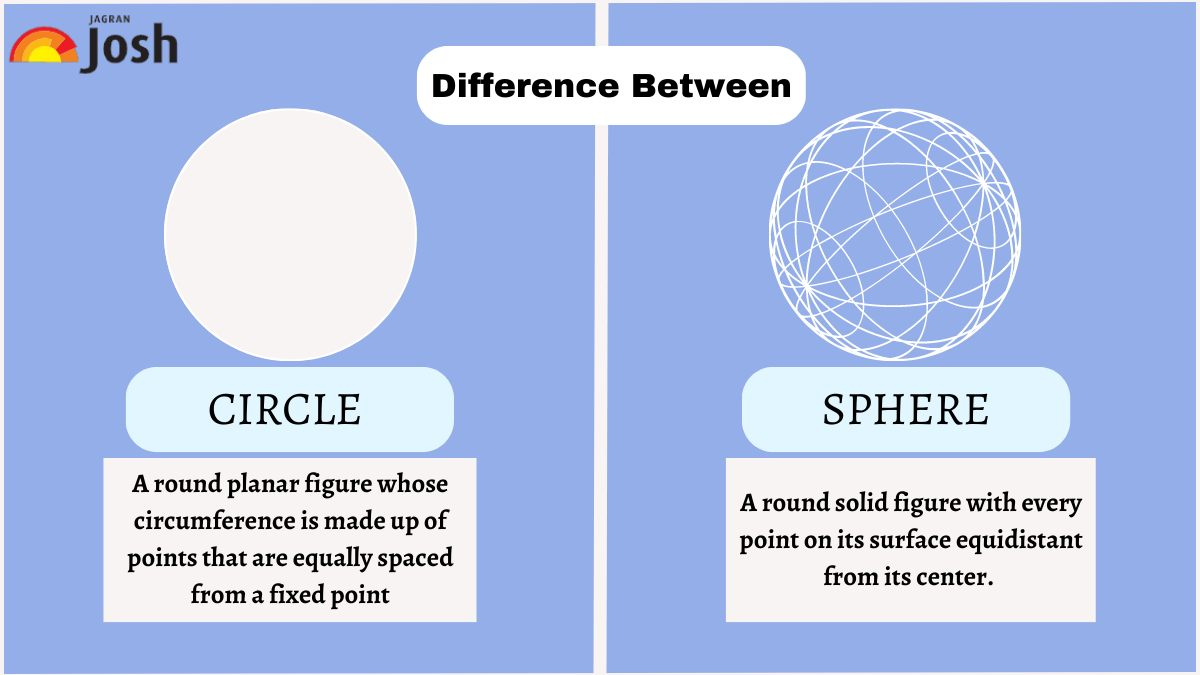# What Is The Difference Between Circle And Sphere?

A circle and a sphere are two geometric shapes that are entirely different. This article will look at the differences between a circle and a sphere and their individual formulas.What Is The Difference Between Circle And Sphere?

Circles and spheres are two geometric figures that share a few similarities yet also have distinct characteristics that set them apart. They are different in a lot of ways. In this article, we'll look at circles and spheres to learn what they are, how they're calculated, and how they differ from one another.

## What is a circle?

A circle is a two-dimensional figure that is defined by a set of points that are all the same distance from a given point, known as the center. It is considered a type of line with a collection of points and has the property that every point along the line is uniformly spaced from a fixed point on a plane. It is made up of a closed loop that separates its planes into inner and outer sections.

Also Read | What Is The Difference Between Black Holes And Wormholes?

The center, circumference, tangent, and chord are some of a circle's many characteristics. The lines that connect the radius to its center represent the radius of that specific circular line, and the letter "r" indicates the radius's length.

## What is a sphere?

A sphere is a three-dimensional version of a circle, with all points on its surface being equidistant from the center. A sphere is also composed of a circumference, tangent, and chord, as well as many other characteristics that are related to the three-dimensional shape. The diameter of the sphere is defined as the line joining the far-off edge points. The sphere has no circumference at all. The sphere contains little hemispheres and circles.

Also Read | What Is The Difference Between Resume And CV?

### How to calculate the area and volume of circles and spheres?

Area of a Circle = π r2

Area of a Sphere = 4 π r2

A circle has no volume since it is a two-dimensional figure.

Volume of a Sphere = 4/3π r3

Also Read | What Is The Difference Between Reversible and Irreversible Reaction?

## Difference between Circle and Sphere

 Circle Sphere Definition A planar figure that has all of its points equally spaced from the center. A completely round and solid figure in which every point on its surface is equally spaced from the center. Dimension It is a two-dimensional figure. It is a three–dimensional figure. Components Only its surface area can be calculated. Both surface area and volume can be calculated. Area π r² 4 π r² Volume No volume Volume = 4/3 π r³ Circumference 2 π r No circumference Equation (x−a)2+(y−b)2= r2 (x−h)2+(y−k)2+(z−l)2=r2

To sum it up, though both being round in shape, circles and spheres are different in every other way. While circles are two-dimensional figures that can be measured by their circumference, spheres are three-dimensional and require the measurement of their volume.

Also Read | What Is The Difference Between Reflex Action And Walking?

Get the latest General Knowledge and Current Affairs from all over India and world for all competitive exams.
खेलें हर किस्म के रोमांच से भरपूर गेम्स सिर्फ़ जागरण प्ले पर